## Tuesday, 26 March 2013

### Minecraft: Pi Edition - Create Massive 3D Models

I love a massive construction in Minecraft, you know when someone creates a scale model of the earth or a house to put the Taj Mahal to shame, but I'm lazy and I'm definitely not going to spend months of my time building one.  What about a program that builds them for you.

What about a program that takes a 3d model, runs through all those points and polygons and draws a representation of the model in Minecraft.

3D Models in Minecraft

Update - Ive pushed the boundaries of this program much further and rendered Manhattan Island in Minecraft.

How
The concept of making this work is pretty simple.

3d models can be saved as a particular type of file, an obj, which is an uncompressed text representation of the model, it describes all the points (aka vertices) as x, y, z coordinates and faces, 3 or more points which when connected create a block or polygon.

My program reads these obj files and then creates line between the points of the faces, effectively creating a wire frame representation of the model in Minecraft.  Simple eh!

The models need to be scaled appropriately to be able to fit in the minecraft world, the scales depends on the detail within the model .

At the moment the program only uses the vertices and faces within the obj files, but it certainly could be extended to create filled polygons rather than wireframe, cope with curves and potentially to use different surfaces and textures.

Update - I have created version 2 of this program, which draws filled polygons and uses the materials within obj files to pick an appropriate block, allowing the model to now be in 'colour'.A Raspberry Pi rendered in Minecraft

You can download the code direct from git-hub, so run minecraft, open/create a world and follow the instructions:

sudo apt-get install git-core
cd ~
git clone https://github.com/martinohanlon/minecraft-renderObj.git
cd minecraft-renderObj
python minecraft-renderObj.py

Code
I've included the code here for reference, but if you want to get it working I suggest you download the repository from github using the instructions above as you will also need other files (e.g. obj's) in to get the code working.

```#www.stuffaboutcode.com
#Raspberry Pi, Minecraft - Create 3D Model from Obj file

#import the minecraft.py module from the minecraft directory
import minecraft.minecraft as minecraft
#import minecraft block module
import minecraft.block as block
#import time, so delays can be used
import time

# return maximum of 2 values
def MAX(a,b):
if a > b: return a
else: return b

# return step
def ZSGN(a):
if a < 0: return -1
elif a > 0: return 1
elif a == 0: return 0

# draw point
def point3d(mc, x, y, z, blockType):
mc.setBlock(x,y,z,blockType)

# draw a line in 3d space
def line3d(mc, x1, y1, z1, x2, y2, z2, blockType):
dx = x2 - x1
dy = y2 - y1
dz = z2 - z1

ax = abs(dx) << 1
ay = abs(dy) << 1
az = abs(dz) << 1

sx = ZSGN(dx)
sy = ZSGN(dy)
sz = ZSGN(dz)

x = x1
y = y1
z = z1

# x dominant
if (ax >= MAX(ay, az)):
yd = ay - (ax >> 1)
zd = az - (ax >> 1)
loop = True
while(loop):
point3d(mc, x, y, z, blockType)
if (x == x2):
loop = False
if (yd >= 0):
y += sy
yd -= ax
if (zd >= 0):
z += sz
zd -= ax
x += sx
yd += ay
zd += az
# y dominant
elif (ay >= MAX(ax, az)):
xd = ax - (ay >> 1)
zd = az - (ay >> 1)
loop = True
while(loop):
point3d(mc, x, y, z, blockType)
if (y == y2):
loop=False
if (xd >= 0):
x += sx
xd -= ay
if (zd >= 0):
z += sz
zd -= ay
y += sy
xd += ax
zd += az
# z dominant
elif(az >= MAX(ax, ay)):
xd = ax - (az >> 1)
yd = ay - (az >> 1)
loop = True
while(loop):
point3d(mc, x, y, z, blockType)
if (z == z2):
loop=False
if (xd >= 0):
x += sx
xd -= az
if (yd >= 0):
y += sy
yd -= az
z += sz
xd += ax
yd += ay

V = [] #vertex
T = [] #texcoords
N = [] #normals
F = [] #face indexies

fh = open(filename)
for line in fh :
if line == '#' : continue
line = line.strip().split(' ')
if line == 'v' : #vertex
V.append(line[1:])
elif line == 'vt' : #tex-coord
T.append(line[1:])
elif line == 'vn' : #normal vector
N.append(line[1:])
elif line == 'f' : #face
face = line[1:]
for i in range(0, len(face)) :
face[i] = face[i].split('/')
# OBJ indexies are 1 based not 0 based hence the -1
# convert indexies to integer
for j in range(0, len(face[i])) :
if face[i][j] != "":
face[i][j] = int(face[i][j]) - 1

F.append(face)

return V, T, N, F

# strips the x,y,z co-ords from a vertex line, scales appropriately, rounds and converts to int
def getVertexXYZ(vertexLine, scale, startCoord, swapYZ):
# convert, round and scale
x = int((float(vertexLine) * scale) + 0.5)
y = int((float(vertexLine) * scale) + 0.5)
z = int((float(vertexLine) * scale) + 0.5)
x = x + startCoord.x
y = y + startCoord.y
z = z + startCoord.z
# swap y and z coord if needed
if swapYZ == True:
swap = y
y = z
z = swap
return x, y, z

# main program
if __name__ == "__main__":

# COORDSSCALE = factor to scale the co-ords by
# STARTCOORD = where to start the model, the relative position 0
# CLEARAREA1/2 = 2 points the program should clear an area in between to put the model in
# SWAPYZ = True to sway the Y and Z dimension
# BLOCKTYPE = type of block to build the model in

# Cube
#COORDSSCALE = 10
#STARTCOORD = minecraft.Vec3(0,10,0)
#BLOCKTYPE = block.STONE
#SWAPYZ = False

# Shuttle
#COORDSSCALE = 4
#STARTCOORD = minecraft.Vec3(-60,0,20)
#CLEARAREA1 = minecraft.Vec3(-30, 5, -30)
#CLEARAREA2 = minecraft.Vec3(-90, 30, 30)
#BLOCKTYPE = block.WOOL
#SWAPYZ = True

# Shyscraper
COORDSSCALE = 1.4
STARTCOORD = minecraft.Vec3(0,10,15)
CLEARAREA1 = minecraft.Vec3(-30, -3, -15)
CLEARAREA2 = minecraft.Vec3(30, 65, 35)
BLOCKTYPE = block.IRON_BLOCK
SWAPYZ = False

#COORDSSCALE = 3
#STARTCOORD = minecraft.Vec3(0,-431,-60)
#CLEARAREA1 = minecraft.Vec3(-30, -30, -30)
#CLEARAREA2 = minecraft.Vec3(30, 65, -110)
#BLOCKTYPE = block.GOLD_BLOCK
#SWAPYZ = False

# Cessna
#COORDSSCALE = 2
#STARTCOORD = minecraft.Vec3(-75, 25, -60)
#CLEARAREA1 = minecraft.Vec3(-30, 15, -30)
#CLEARAREA2 = minecraft.Vec3(-100, 65, -90)
#BLOCKTYPE = block.WOOD_PLANKS
#SWAPYZ = False

#Connect to minecraft by creating the minecraft object
# - minecraft needs to be running and in a game
mc = minecraft.Minecraft.create()

#Post a message to the minecraft chat window
mc.postToChat("Hi, Minecraft 3d model maker, www.stuffaboutcode.com")

# clear a suitably large area
mc.setBlocks(CLEARAREA1.x, CLEARAREA1.y, CLEARAREA1.z, CLEARAREA2.x, CLEARAREA2.y, CLEARAREA2.z, block.AIR)
time.sleep(3)

# loop through faces
for face in faces:
#persist the first vertex of the face
firstVertex = face
#get the x,y,z co-ords of the first vertex
firstVertexX, firstVertexY, firstVertexZ = getVertexXYZ(vertices[firstVertex], COORDSSCALE, STARTCOORD, SWAPYZ)
#last vertex is current none
lastVertex = None

# loop through vertex's in face and draw lines between them
for vertex in face:
vertexX, vertexY, vertexZ = getVertexXYZ(vertices[vertex], COORDSSCALE, STARTCOORD, SWAPYZ)

if lastVertex != None:
# got 2 vertices, draw a line between them
line3d(mc, lastVertexX, lastVertexY, lastVertexZ, vertexX, vertexY, vertexZ, BLOCKTYPE)

#persist the last vertex found
lastVertex = vertex
lastVertexX, lastVertexY, lastVertexZ = vertexX, vertexY, vertexZ

# draw a line between the last and first vertex's
line3d(mc, lastVertexX, lastVertexY, lastVertexZ, firstVertexX, firstVertexY, firstVertexZ, BLOCKTYPE)

I got a number of obj files from this website - http://people.sc.fsu.edu/~jburkardt/data/obj/obj.html

I got the basic code to parse an obj file here - http://programminglinuxgames.blogspot.co.uk/2010/09/parsing-wavefront-obj-file-format-using.html

I used this C code as the basis for my python function to draw line in 3d - http://www.luberth.com/plotter/line3d.c.txt.html

Short link to this post - http://bit.ly/14mzPXY

1.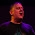That is AWESOME, Martin. Nicely done. Blogged about it here: http://www.recantha.co.uk/blog/?p=3228
Thanks for sharing the script - looks like a lot of fun :-)

2.mmmm, I'm just wondering if with a bit (lot) of messing, if this could be done in reverse, so minecraft could be used as a 3d modeling environment, and *.obj files could be produced from a minecraft build.

hehe, Time to start playing.

1.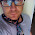It sounds 'possible', Im not sure how you would determine a face or a polygon within the minecraft world as basically everything is blocks but you could certainly make a script that creates an obj file every block in a certain region. Fancy having a go?

2.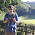I know you can use mineways or jMc2Obj to convert a minecraft world to .obj format like you want but I only know about windows

3.Martin, fantastic script and idea.

Just wondering what software you used other than Sketchup to 'scale' your models as you mentioned. I've worked out how to export from Sketchup as OBJ, but not sure where to go from there.

Am I right in thinking you just call the script and tell it which .obj to render e.g.

python minecraft-renderObj.py shuttle.obj

I'd love to get this up and running so I can do show and tell at school.

1.The models are scaled by the program itself.

In order to get the program working with a new obj file, you just need to configure a number of CONSTANTS at the start of the program (this is what they are for the cube:

COORDSSCALE = 10
STARTCOORD = minecraft.Vec3(0,10,0)
BLOCKTYPE = block.STONE
SWAPYZ = False

The COORDSCALE constant is how you scale the model, so in the case of the cube, each vertice (x,y,z point) in the model is multiplied by 10. I would either open the obj file in notepad and have a look at the vertices or just use or 'try it' with a scale of 1, if its too big, scale it down by using 0.1, if its too small scale it up to 10.

4.Ignore my stupidity, I went for a noodle round the code and worked out it just rendered what you put in the code. However in your excellent video you demonstrate looking at your models on the PI in some sort of 3D viewer, could you tell me what software you were using for that?

1.When I show them in my videos, they are just pictures, I just use the standard image view app which comes installed with raspbian.

5.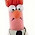Very cool script! I'm going to get my pi cranking out an island right now. Thank you for the information, really appreciate the work.

6.whenever I create it it spawns me in like the air and im trapped . what to I do

1.Im not really sure I understand your problem.

The script doesnt change the players position. If you find yourself trapped you could use the API to move the player to any position using mc.player.setPos(x,y,z)

7.how do you install this on a windows based minecraft

1.you will need the Raspberry Juice plugin, which emulates the pi api on the PC version of Minecraft

8.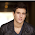Nice tutorial. Give an example for how the game is very profitable. It is very popular right now. I like it. But I've been advised to this program 3d-kstudio.com/product/trackscripts/ . Don't tell me it's good?

9.Hello Martin,

I m looking for a method to export a creation .obj or .stl for 3d printing ?